# 2. Simple optimisation problem#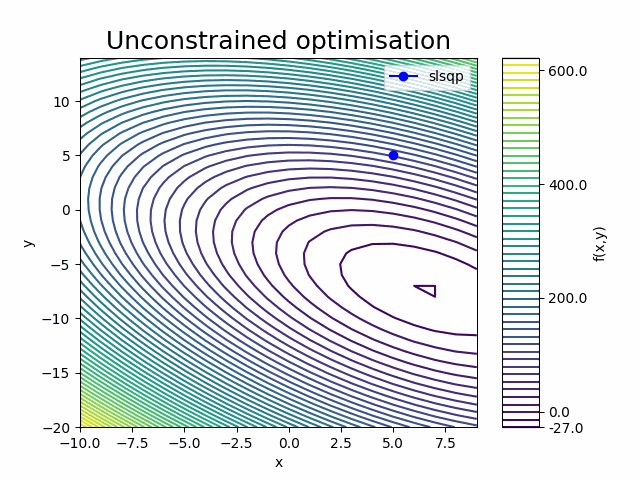Duration: 15 min

In this example we solve a simple analytical function minimisation problem. We create an optimisation driver component using the openMDAO python package, and then use it to determine the unconstrained and constrained minimum values of the paraboloid function from the previous example.

## 2.1. Opening a saved session#

Since we already created and analysed the paraboloid component previously, we can load our previous session to speed things up.

Select Open from the interface controls to load the JSON formatted version of our previous session (dapta_input.json). Alternatively, copy the object below into a text editor and save it locally, then select Open to load it.

{
"components": [
{
"name": "paraboloid",
"api": "generic-python3-comp:latest",
"options": {},
"parameters": {
"user_input_files": [],
"x": 5.0,
"y": 5.0,
"f_xy": 0.0
},
"inputs": {
"x": "default",
"y": "default"
},
"outputs": {
"f_xy": "default"
}
}
],
"connections": [],
"workflow": {
"start": "paraboloid",
"end": "paraboloid"
}
}


The paraboloid component should have appeared in the workspace, but the question mark next to the component name indicates that it is missing some data. To fully define it, we need to upload the component setup.py and compute.py input files under the component Properties tab again. The file contents are available under the Simple Component Analysis example.

## 2.2. Create the driver component#

Right-click in the workspace and select Add Empty Node. This adds an empty template component to your workspace.

Select the empty component to edit it.

### 2.2.1. Properties#

In the Properties tab, fill in the component name, open-mdao, and select the driver component API generic-python3-driver:latest.

Just as in the previous example, the python driver requires setup.py and compute.py input files to be uploaded. In addition, we also upload a requirements.txt file, so that we can access the openMDAO package in the python code. You can inspect the contents of the files below.

In the compute.py file, we can see that the imports include the ‘numpy’ and ‘openmdao’ packages that we listed in the requirements.txt file. In addition, we import a call_compute function from the component_api2.py module that is specific to the generic-python3-driver API and allows the driver to execute other components (this is the main difference with the generic-python3-comp API!).

The last import in the compute.py module (from om_component import ...) is specific to our component and provides a custom implementation of the openMDAO ExplicitComponent class. The contents of the om_component.py module are shown below and we will upload this as a Parameter input file in the next section.

Finally, check the box next to the Driver option as shown below.

from datetime import datetime
from pathlib import Path

def setup(
inputs: dict = {"design": {}, "implicit": {}, "setup": {}},
outputs: dict = {"design": {}, "implicit": {}, "setup": {}},
parameters: dict = {
"user_input_files": [],
"inputs_folder_path": "",
"outputs_folder_path": "",
},
) -> dict:
"""Editable setup function."""

if "driver" not in parameters:
# assume we want to run an optimisation with default settings
parameters["driver"] = {"type": "optimisation"}

message = f"{datetime.now().strftime('%Y%m%d-%H%M%S')}: Setup completed."

return {"message": message, "parameters": parameters}

from datetime import datetime
from pathlib import Path
import traceback
from contextlib import redirect_stdout
import numpy as np
import json
from copy import deepcopy

import openmdao.api as om
from matplotlib import pyplot as plt  # type: ignore

from om_component import OMexplicitComp, OMimplicitComp  # type: ignore

OM_DEFAULTS = {
"nonlinear_solver": {
"class": om.NewtonSolver,
"kwargs": {"solve_subsystems": False},
},
"linear_solver": {
"class": om.DirectSolver,
"kwargs": {},
},
}

def compute(
inputs: dict = {"design": {}, "implicit": {}, "setup": {}},
outputs: dict = {"design": {}, "implicit": {}, "setup": {}},
partials: dict = {},
options: dict = {},
parameters: dict = {
"user_input_files": [],
"inputs_folder_path": "",
"outputs_folder_path": "",
},
) -> dict:

"""Editable compute function."""

print("OpenMDAO problem setup started.")

workflow = parameters["workflow"]
run_folder = Path(parameters["outputs_folder_path"])
all_connections = parameters.get("all_connections", [])

# 1) define the simulation components
prob = om.Problem()

groups = {}
if "Groups" in parameters:
for group in parameters["Groups"]:
name = reformat_compname(group["name"])
kwargs = group.get("kwargs", {})
name,
om.Group(),
**kwargs,
)
if "solvers" in group:
for solver in group["solvers"]:
if solver["type"] == "nonlinear_solver":
groups[name].nonlinear_solver = OM_DEFAULTS["nonlinear_solver"][
"class"
](**OM_DEFAULTS["nonlinear_solver"]["kwargs"])
solver_obj = groups[name].nonlinear_solver
elif solver["type"] == "linear_solver":
groups[name].linear_solver = OM_DEFAULTS["linear_solver"][
"class"
](**OM_DEFAULTS["linear_solver"]["kwargs"])
solver_obj = groups[name].nonlinear_solver
else:
raise ValueError(
f"Solver of type {solver['type']} is not implemented."
)
if "options" in solver:
for option, val in solver["options"].items():
if option in ["iprint", "maxiter"]:
solver_obj.options[option] = int(val)
else:
solver_obj.options[option] = val

def get_comp_by_name(name, objs: dict):

comp_type_lookup = {
"ExplicitComponents": OMexplicitComp,
"ImplicitComponents": OMimplicitComp,
}

for key, obj in objs.items():
filtered = [comp_obj for comp_obj in obj if comp_obj["name"] == name]
if filtered:
return [comp_type_lookup[key], filtered]
return OMexplicitComp, None  # default

model_lookup = {}
for component in workflow:
# defaults
kwargs = {}
fd_step = 0.1
model = prob.model
has_compute_partials = True  # set this to False if fd gradients should be used

objs = {
k: parameters[k]
for k in ["ExplicitComponents", "ImplicitComponents"]
if k in parameters
}
comp_type, comp_obj = get_comp_by_name(component, objs)
if comp_obj:
kwargs = comp_obj.get("kwargs", kwargs)
fd_step = comp_obj.get("fd_step", fd_step)
has_compute_partials = comp_obj.get(
"has_compute_partials", has_compute_partials
)
model = groups.get(comp_obj.get("group"), model)

model_lookup[component] = model
reformat_compname(component),
comp_type(
compname=component,
fd_step=fd_step,
has_compute_partials=has_compute_partials,
),
**kwargs,
)

if "ExecComps" in parameters and parameters["ExecComps"]:
for component in parameters["ExecComps"]:
reformat_compname(component["name"]),
om.ExecComp(component["exprs"]),
**component["kwargs"],
)

# 2) define the component connections
def get_var_str(c, name):
return f"{reformat_compname(c)}.{name.replace('.','-')}"

for connection in all_connections:
if connection["type"] == "design":
prob.model.connect(
get_var_str(connection["origin"], connection["name_origin"]),
get_var_str(connection["target"], connection["name_target"]),
)

if parameters["driver"]["type"] == "optimisation":
# 3) setup the optimisation driver options
prob.driver = om.ScipyOptimizeDriver()
prob.driver.options["optimizer"] = parameters["optimizer"]
prob.driver.options["maxiter"] = parameters["max_iter"]
prob.driver.options["tol"] = parameters["tol"]
prob.driver.opt_settings["disp"] = parameters["disp"]
prob.driver.options["debug_print"] = parameters["debug_print"]

if "approx_totals" in parameters and parameters["approx_totals"]:
# ensure FD gradients are used
prob.model.approx_totals(
method="fd", step=parameters["fd_step"], form=None, step_calc=None
)

elif parameters["driver"]["type"] == "doe":
# 3) alternative: setup a design of experiments

levels = parameters["driver"]["kwargs"].get("levels", 2)
if isinstance(levels, float):  # All have the same number of levels
levels = int(levels)
elif isinstance(levels, dict):  # Different DVs have different number of levels
levels = {k: int(v) for k, v in levels.items()}

prob.driver = DOEDriver(
om.FullFactorialGenerator(levels=levels),
reset_vars=parameters["driver"]["kwargs"].get("reset_vars", {}),
store_case_data=parameters["driver"]["kwargs"].get("store_case_data", {}),
store_parameters=parameters["driver"]["kwargs"].get("store_parameters", {}),
run_folder=run_folder,
)

if "input_variables" in parameters:
for var in parameters["input_variables"]:
upper = var["upper"]
lower = var["lower"]
if "component" in var:
comp_obj = reformat_compname(var["component"])
f"{comp_obj}.{var['name'].replace('.', '-')}",
lower=lower,
upper=upper,
)
else:
var["name"].replace(".", "-"), lower=lower, upper=upper
)
val_default = var.get("value", lower)
prob.model.set_input_defaults(
var["name"].replace(".", "-"), val_default
)

# 5) add an objective and constraints
if "output_variables" in parameters:
for var in parameters["output_variables"]:
comp_obj = reformat_compname(var["component"])
name = f"{comp_obj}.{var['name'].replace('.', '-')}"

# set scaling from parameter input file
scaler = var.get("scaler", None)

if var["type"] == "objective":
elif var["type"] == "constraint":
lower = var.get("lower", None)
upper = var.get("upper", None)
)

prob.setup()  # required to generate the n2 diagram
print("OpenMDAO problem setup completed.")

if "visualise" in parameters and "n2_diagram" in parameters["visualise"]:
# save n2 diagram in html format
om.n2(
prob,
outfile=str(run_folder / "n2.html"),
show_browser=False,
)

if parameters["driver"]["type"] == "optimisation":
dict_out = run_optimisation(prob, parameters, run_folder)

# elif parameters["driver"]["type"] == "check_partials":
#     dict_out = run_check_partials(prob, parameters)

# elif parameters["driver"]["type"] == "check_totals":
#     dict_out = run_check_totals(prob, parameters)

elif parameters["driver"]["type"] == "doe":

# elif parameters["driver"]["type"] == "post":
#     dict_out = run_post(prob, parameters)

else:
with open(run_folder / "trim_convergence.log", "w") as f:
with redirect_stdout(f):
prob.run_model()
dict_out = {}

message = f"{datetime.now().strftime('%Y%m%d-%H%M%S')}: OpenMDAO compute completed."
print(message)

if dict_out:
outputs["design"] = dict_out

return {"message": message, "outputs": outputs}

def run_optimisation(prob, parameters, run_folder):

# 6) add a data recorder to the optimisation problem
r_name = str(
run_folder
/ (
"om_problem_recorder_"
+ datetime.now().strftime("%Y%m%d-%H%M%S")
+ ".sqlite"
)
)
r = om.SqliteRecorder(r_name)
prob.driver.recording_options["record_derivatives"] = True

# setup the problem again
prob.setup()

if "visualise" in parameters and "scaling_report" in parameters["visualise"]:
# NOTE: running the model can generate large large amounts of stored data in orchestrator, which
# can cause prob.setup() to fail if it is called again, so only execute
# prob.run_model() after all setup has been completed
with open(run_folder / "scaling_report.log", "w") as f:
with redirect_stdout(f):
prob.run_model()
prob.driver.scaling_report(
outfile=str(run_folder / "driver_scaling_report.html"),
title=None,
show_browser=False,
jac=True,
)

# 7) execute the optimisation
try:
with open(run_folder / "run_driver.log", "w") as f:
with redirect_stdout(f):
prob.run_driver()
except Exception as e:
print(f"run driver exited with error: {e}")
tb = traceback.format_exc()
raise ValueError("OpenMDAO Optimisation error: " + tb)

opt_output = {}
# print("Completed model optimisation - solution is: \n inputs= (")
for var in parameters["input_variables"]:
name = var["name"]
# print(
#     f"{comp}.{name}: "
#     + str(prob.get_val(f"{comp}.{name.replace('.', '-')}"))
#     + " "
# )
if "component" in var:
comp = var["component"]
opt_output[f"{comp}.{name}"] = prob.get_val(
f"{reformat_compname(comp)}.{name.replace('.', '-')}"
).tolist()
else:
opt_output[name] = prob.get_val(name.replace(".", "-")).tolist()
# print("), \n outputs = (")
for var in parameters["output_variables"]:
comp = var["component"]
name = var["name"]
# print(
#     f"{comp}.{name}: "
#     + str(prob.get_val(f"{comp}.{name.replace('.', '-')}"))
#     + " "
# )
opt_output[f"{comp}.{name}"] = prob.get_val(
f"{reformat_compname(comp)}.{name.replace('.', '-')}"
).tolist()
# print(")")

print(opt_output)

if "visualise" in parameters and "plot_history" in parameters["visualise"]:
post_process_optimisation(parameters, run_folder, r_name)

return opt_output

# 7) execute the driver in parallel
prob_copy = deepcopy(prob)
print(f"problem id for color {color}: ", id(prob_copy))

# set driver instance properties
prob_copy.driver.color = color
try:
prob_copy.run_driver()
except Exception as e:
print(f"run driver exited with error: {e}")
tb = traceback.format_exc()
return f"OpenMDAO DOE error: {tb}"

with open(run_folder / f"run_driver.log", "w") as f:
with redirect_stdout(f):
errors = list(msgs)
if errors and errors:
raise ValueError(errors)

if "visualise" in parameters and "plot_history" in parameters["visualise"]:
from post import post_process_doe

post_process_doe(
parameters,
run_folder,
)

return {}

def reformat_compname(name):
# openmdao doesn't allow "-" character in component names
return name.replace("-", "_")

def post_process_optimisation(
parameters, run_folder, r_name, only_plot_major_iter=True
):
# Isolate "problem" as your source
driver_cases = cr.list_cases("driver", out_stream=None)

# plot the iteration history from the recorder data
inputs_history = {
key: []
for key in [
f"{reformat_compname(var['component'])}.{var['name'].replace('.', '-')}"
for var in parameters["input_variables"]
]
}
outputs_history = {
key: []
for key in [
f"{reformat_compname(var['component'])}.{var['name'].replace('.', '-')}"
for var in parameters["output_variables"]
]
}
for key in driver_cases:
case = cr.get_case(key)
if (only_plot_major_iter and case.derivatives) or not only_plot_major_iter:
# get history of inputs
for key in inputs_history:
inputs_history[key].append(case.outputs[key])
# get history of outputs
for key in outputs_history:
outputs_history[key].append(case.outputs[key])

# plot output in userfriendly fashion
_plot_iteration_histories(
inputs_history=inputs_history,
outputs_history=outputs_history,
run_folder=run_folder,
)

def _plot_iteration_histories(
inputs_history=None, outputs_history=None, run_folder=None
):
# plot input histories
for key in inputs_history:
input_data = inputs_history[key]
input_data = np.array(input_data)
iterations = range(input_data.shape)

plt.figure()
for data_series in input_data.T:
plt.plot(iterations, data_series, "-o")
plt.grid(True)
plt.title(key)
plt.savefig(str(run_folder / (key + ".png")))

# plot output histories
for key in outputs_history:
output_data = outputs_history[key]
output_data = np.array(output_data)
iterations = range(output_data.shape)

plt.figure()
for data_series in output_data.T:
plt.plot(iterations, data_series, "-o")
plt.grid(True)
plt.title(key)
plt.savefig(str(run_folder / (key + ".png")))

plt.show()

class DOEDriver(om.DOEDriver):
def __init__(
self,
generator=None,
reset_vars: dict = None,
store_case_data: list = None,
store_parameters: dict = None,
run_folder: Path = None,
**kwargs,
):
self.reset_vars = reset_vars
self.cases_store = []
self.store_case_data = store_case_data
self.store_parameters = store_parameters
self.run_folder = run_folder
self.color = 0
super().__init__(generator=generator, **kwargs)

def run(self):
"""
Generate cases and run the model for each set of generated input values.

Returns
-------
bool
Failure flag; True if failed to converge, False is successful.
"""
self.iter_count = 0
self._quantities = []

# set driver name with current generator
self._set_name()

dv_meta = self._designvars
self._indep_list = list(dv_meta)

objs = self.get_objective_values()
for name in objs:
self._quantities.append(name)

con_meta = self._cons
for name, _ in con_meta.items():
self._quantities.append(name)

for case in self._parallel_generator(self._designvars, self._problem().model):
self._custom_reset_variables()
self._run_case(case)
self.iter_count += 1
self._custom_store_to_json()

return False

def _custom_reset_variables(self):
# reset the initial variable guesses
for k, v in self.reset_vars.items():
self._problem()[k] = v

def _custom_store_to_json(self):
# store the outputs to the json database
self.cases_store.append(
{
**self.store_parameters,
**{
k.split(".")[-1]: self._problem()[k]
for k in self.store_case_data
},
}
)
# dump to json file
fname = f"results_{self.color}.json"
with open(self.run_folder / fname, "w", encoding="utf-8") as f:
json.dump(self.cases_store, f)

def _parallel_generator(self, design_vars, model=None):
"""

Parameters
----------
design_vars : dict
Dictionary of design variables for which to generate values.

model : Group
The model containing the design variables (used by some generators).

Yields
------
list
list of name, value tuples for the design variables.
"""
color = self.color

generator = self.options["generator"]
for i, case in enumerate(generator(design_vars, model)):
if i % size == color:
yield case

if __name__ == "__main__":
with open("open-mdao-driver/parameters.json", "r") as f:
parameters["all_connections"] = [
{
"origin": "vspaero",
"name_origin": "CL",
"target": "trim",
"name_target": "CL",
"type": "design",
},
{
"origin": "vspaero",
"name_origin": "CMy",
"target": "trim",
"name_target": "CMy",
"type": "design",
},
]
parameters["workflow"] = ["vspaero", "trim"]  # defined by controller
parameters["outputs_folder_path"] = "outputs"  # defined by component generic api
compute(
inputs={},
outputs={"design": {}},
partials=None,
options=None,
parameters=parameters,
)

numpy==1.23.5
openmdao==3.16.0
matplotlib==3.7.0
fluids==1.0.22
pandas==1.5.3

""" Optimisation Component classes for OpenMDAO and associated utilities."""

import numpy as np
import openmdao.api as om  # type: ignore

from component_api2 import call_compute, call_setup
import traceback

class OMexplicitComp(om.ExplicitComponent):
"""standard component that follows the OM conventions"""

def __init__(self, compname, fd_step, has_compute_partials=True):
super().__init__()
self.compname = compname
self.iter = 0
self.partial_dict = None
self.fd_step = fd_step
self._has_compute_partials = has_compute_partials  # overrides parent class attribute with user defined parameter

def setup(self):

message = {"component": self.compname}
_, component_dict = call_setup(message)
inputs = component_dict["input_data"]["design"]
outputs = component_dict["output_data"]["design"]

# initialise the inputs
if inputs:
for variable in inputs:

# initialise the outputs
if outputs:
for variable in outputs:

def setup_partials(self):
# Get the component partial derivative information

message = {"component": self.compname}
_, component_dict = call_setup(message)

if "partials" in component_dict and component_dict["partials"]:
self.partial_dict = component_dict["partials"]
for resp, vars in self.partial_dict.items():
for var, vals in vars.items():
self.declare_partials(
resp.replace(".", "-"), var.replace(".", "-"), **vals
)
else:
# calculate all paritials using finite differencing
self.declare_partials("*", "*", method="fd", step=self.fd_step)

def compute(self, inputs, outputs, discrete_inputs=None, discrete_outputs=None):

print("Calling compute.")
# calculate the outputs
# Note: transform all np.ndarrays into nested lists to allow formatting to json
input_dict = {"design": reformat_inputs(inputs._copy_views())}

message = {
"component": self.compname,
"inputs": input_dict,
"get_outputs": True,
}
print("message: \n", str(message))

try:
_, data = call_compute(message)
if not "outputs" in data:
raise ValueError(f"Error: Compute output missing - output was: {data}.")
except Exception as e:
print(f"Compute of {self.compname} failed, input data was: {str(message)}")
tb = traceback.format_exc()
print(tb)
raise ValueError(
f"OM Explicit component {self.compname} compute error: " + tb
)

val_outputs = data["outputs"]["design"]

# OpenMDAO doesn't like the outputs dictionary to be overwritten, so
for output in outputs:
outputs[output] = val_outputs[output.replace("-", ".")]

def compute_partials(self, inputs, J):
"""Jacobian of partial derivatives."""

print("Calling compute_partials.")

self.iter += 1
input_dict = reformat_inputs(inputs._copy_views())

message = {
"component": self.compname,
"inputs": input_dict,
"get_outputs": False,
}
print("message: \n", str(message))

try:
_, data = call_compute(message)
if not "partials" in data:
raise ValueError(
f"Error: Compute partial derivatives missing - output was: {data}."
)
self.partial_dict = data["partials"]
except Exception as e:
print(
f"Compute partials of {self.compname} failed, input data was: {str(message)}"
)
tb = traceback.format_exc()
print(tb)
raise ValueError(
f"OM Explicit component {self.compname} compute error: " + tb
)

if self.partial_dict:
for resp, vars in self.partial_dict.items():
for var, vals in vars.items():
if "val" in vals:
J[resp.replace(".", "-"), var.replace(".", "-")] = vals["val"]
# print(dict(J))
else:
raise ValueError(f"Component {self.compname} has no Jacobian defined.")

class OMimplicitComp(om.ImplicitComponent):
"""standard implicit component that follows the OM conventions"""

def __init__(self, compname, fd_step, has_compute_partials=False):
super().__init__()
self.compname = compname
self.iter = 0
self.partial_dict = None
self.fd_step = fd_step

def setup(self):

message = {"component": self.compname}
_, component_dict = call_setup(message)
inputs = component_dict["input_data"]["design"]
outputs = component_dict["output_data"]["design"]

# initialise the inputs
if inputs:
for variable in inputs:

# initialise the outputs
if outputs:
for variable in outputs:

def setup_partials(self):

message = {"component": self.compname}
_, component_dict = call_setup(message)

if "partials" in component_dict and component_dict["partials"]:
self.partial_dict = component_dict["partials"]
for resp, vars in self.partial_dict.items():
for var, vals in vars.items():
self.declare_partials(
resp.replace(".", "-"), var.replace(".", "-"), **vals
)
else:
# calculate all paritials using finite differencing
self.declare_partials("*", "*", method="fd", step=self.fd_step)

def apply_nonlinear(self, inputs, outputs, residuals):

input_dict = {"design": reformat_inputs(inputs._copy_views())}

message = {
"component": self.compname,
"inputs": input_dict,
"get_outputs": True,
}
print("message: \n", str(message))

try:
_, data = call_compute(message)
if not "outputs" in data:
raise ValueError(f"Error: Compute output missing - output was: {data}.")
except Exception as e:
print(f"Compute of {self.compname} failed, input data was: {str(message)}")
tb = traceback.format_exc()
print(tb)
raise ValueError(
f"OM Explicit component {self.compname} compute error: " + tb
)

val_outputs = data["outputs"]["design"]

for output in outputs:
residuals[output] = val_outputs[output.replace("-", ".")]

def reformat_inputs(inputs):
input_dict = inputs
for key in [*input_dict.keys()]:
new_key = key.split(".")[-1].replace("-", ".")
input_dict[new_key] = input_dict.pop(key)
if isinstance(input_dict[new_key], np.ndarray):
input_dict[new_key] = input_dict[new_key].tolist()
return input_dict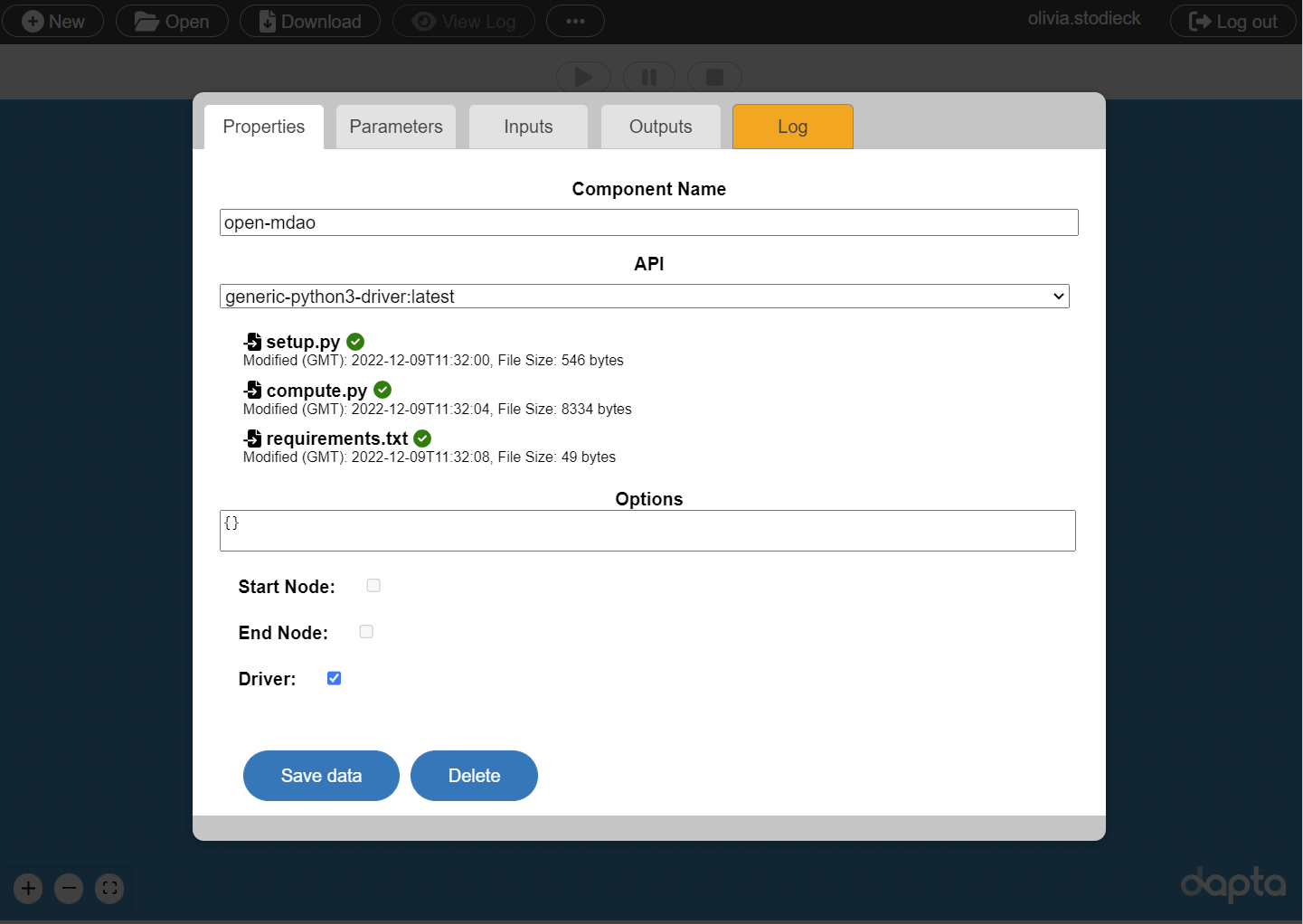### 2.2.2. Parameters#

Select the Parameters tab and copy the ‘unconstrained’ parameters JSON object into the text box.

{
"optimizer": "SLSQP",
"max_iter": 20,
"tol": 1e-8,
"disp": true,
"debug_print": [
"desvars",
"ln_cons",
"nl_cons",
"objs",
"totals"
],
"approx_totals": true,
"fd_step": 0.0001,
"input_variables": [
{
"component": "paraboloid",
"name": "x",
"lower": -50,
"upper": 50
},
{
"component": "paraboloid",
"name": "y",
"lower": -50,
"upper": 50
}
],
"output_variables": [
{
"component": "paraboloid",
"type": "objective",
"name": "f_xy"
}
],
"driver": {
"type": "optimisation"
},
"visualise": [
"n2_diagram"
]
}

{
"optimizer": "SLSQP",
"max_iter": 20,
"tol": 1e-8,
"disp": true,
"debug_print": [
"desvars",
"ln_cons",
"nl_cons",
"objs",
"totals"
],
"approx_totals": false,
"ExplicitComponents": [
{
"name": "paraboloid",
"kwargs": {
"promotes_inputs": [
"x",
"y"
]
}
}
],
"ExecComps": [
{
"name": "constraint",
"exprs": "g = x + y",
"kwargs": {
"promotes_inputs": [
"x",
"y"
]
}
}
],
"input_variables": [
{
"name": "x",
"lower": -50,
"upper": 50,
"value": 5.0
},
{
"name": "y",
"lower": -50,
"upper": 50,
"value": 5.0
}
],
"output_variables": [
{
"component": "paraboloid",
"type": "objective",
"name": "f_xy"
},
{
"component": "constraint",
"type": "constraint",
"name": "g",
"lower": 0.0,
"upper": 10.0
}
],
"driver": {
"type": "optimisation"
},
"visualise": [
"n2_diagram"
]
}


These parameters are used in the compute.py module to define a standard openMDAO optimisation problem.

Next, as mentioned in the previous section, we also need to upload the om_component.py module. Copy the contents of the ‘om_component’ tab above into a text editor and save as ‘om_component.py’.
Select the upload user input files link at the bottom of the Parameters tab to upload the file. The upload was successful if a corresponding entry appears under the ‘user_input_files’ section of the JSON object in the Parameters text box.

By extracting parameters from the python code and defining them in the Parameters tab instead, we can change the optimisation setup without having to view or modify the python code. This makes it easier to track input changes and to compare Runs (e.g. via the session file). The component also becomes more general, robust and re-usable. We will demonstrate the benefits of this approach in the next section by replacing the ‘unconstrained’ parameters with the ‘constrained’ ones.

Select Save data to save the edits and close the component interface.

Select Download from the interface controls to save the current session as a local JSON file.

## 2.3. Executing the driver#

We should now have a valid Run with two components: the paraboloid analysis and the open-mdao driver.

Execute the Run by selecting the play symbol ▶ in the Run controls interface and wait for it to complete. As before, this may take a few minutes as your Run is being processed in the Cloud. If you don’t get a message saying that the Run was successful, try to refresh your web browser page or consult the FAQ section for troubleshooting suggestions.

### 2.3.1. The unconstrained optimisation problem#

We can now inspect the outputs of the unconstrained optimisation Run.

Select View Log to view the Run log as shown below. The optimisation outcome is summarised in the ‘open-mdao’ entry, which indicates that the optimisation completed successfully. The optimisation input values (x, y) converged to the known analytical solution within a few decimals ($$x=\frac{20}{3}$$, $$y=-\frac{22}{3}$$). If we scroll down, we can see that the paraboloid component has 13 separate entries, each one corresponding to one analysis execution of the component.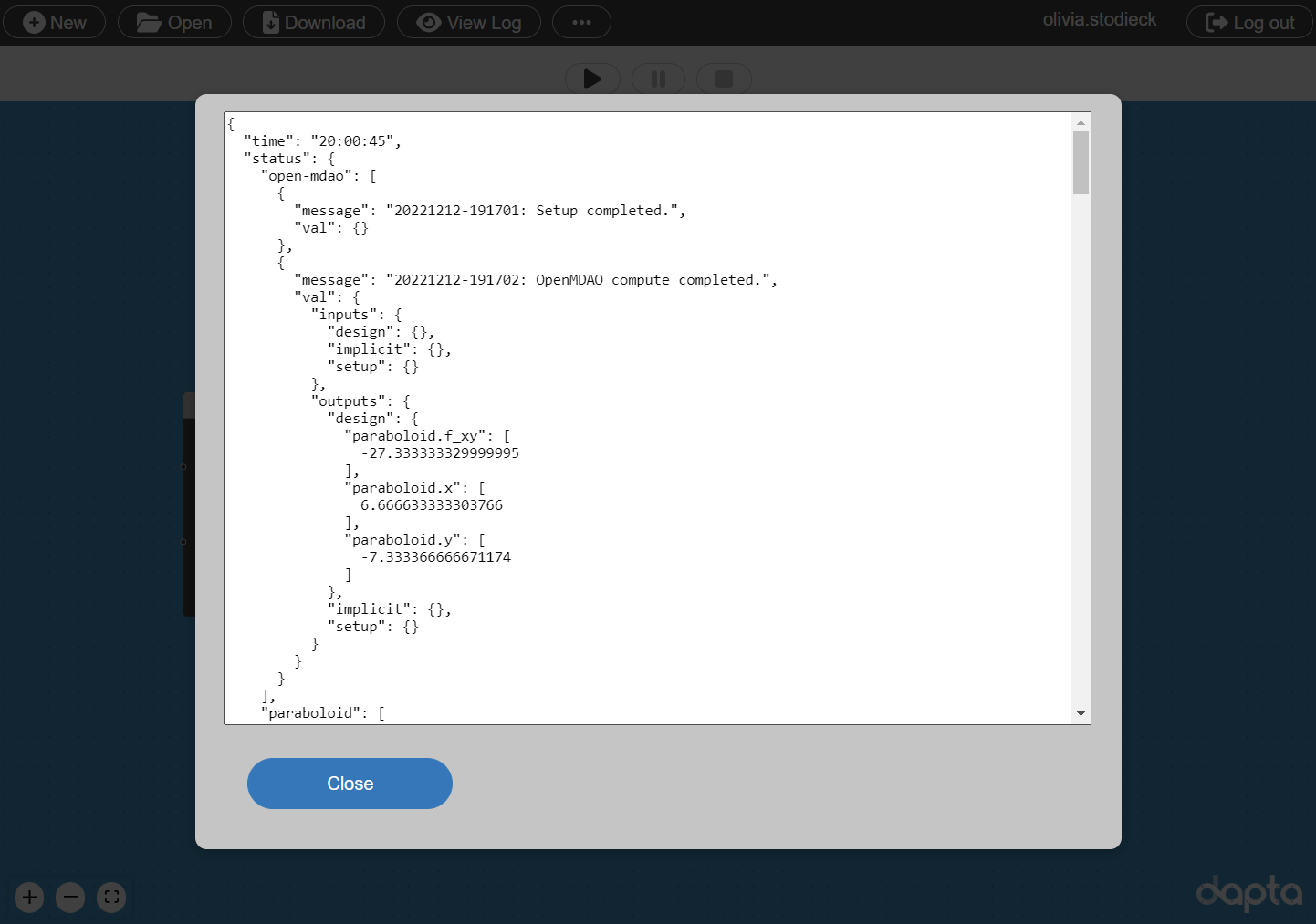We can also look at the outputs of the open-mdao component in more detail. Select the open-mdao component in the workspace and navigate to the Log tab, then select download files snapshot. The ‘outputs’ folder should contain three files:

• n2.html : A visual representation of the optimisation problem. We can switch this output off by removing the ‘n2_diagram’ entry from the ‘visualise’ list in the component Parameters.

• om_problem_recorder_(time-stamp).sqlite : An openMDAO recorder database can be used for further data analysis, visualisation and results storage. The contents of the database can be adjusted (see the use of the ‘om.SqliteRecorder’ class in the open-mdao compute function).

• run_driver.log : a log file that contains the openMDAO stdout output and any additional print() function outputs from the om_component.py module.

Note

Python print() output can be very useful for capturing intermediary variable values and for debugging purposes. In order to access this output in a log file, you can use the following python code construct (see the compute.py module for an example):

from contextlib import redirect_stdout

with open(run_folder / "filename.log", "w") as f:
with redirect_stdout(f):
print("This is a log message!")


Below is an extract from the end of the run_driver.log file. This file provides some interesting insights into the optimisation process.

It appears that the SLSQP optimisation converged to an optimum after 4 iterations, which included 5 ‘Function evaluations’ to calculate the function outputs and 4 ‘Gradient evaluations’ to calculate the derivatives (gradient) of the paraboloid function with respect to the design variables x and y.

Looking at the frequency of the ‘Calling compute’ message in the last iteration, we can see that the ‘Function evaluation’ executed the paraboloid component once and that the ‘Gradient computation’ executed it twice (once for each design variable). This is because we requested the finite difference gradient calculation method by setting "approx_totals": true in the in the Parameters (with the ‘fd_step’ parameter setting the size of the step).

The paraboloid component was therefore executed a total of 13 times, which matches the Run log output.

Save the session data and Run log by selecting Download from the interface controls.

Driver debug print for iter coord: rank0:ScipyOptimize_SLSQP|5
--------------------------------------------------------------
Design Vars
{'paraboloid.x': array([6.66663333]), 'paraboloid.y': array([-7.33336667])}

Calling compute.
message:
{'component': 'paraboloid', 'inputs': {'y': [-7.333366666671174], 'x': [6.666633333303766]}, 'get_grads': False, 'get_outputs': True}
Nonlinear constraints
None

Linear constraints
None

Objectives
{'paraboloid.f_xy': array([-27.33333333])}

Driver total derivatives for iteration: 6
-----------------------------------------

Calling compute.
message:
{'component': 'paraboloid', 'inputs': {'y': [-7.333366666671174], 'x': [6.666733333303766]}, 'get_grads': False, 'get_outputs': True}
Calling compute.
message:
{'component': 'paraboloid', 'inputs': {'y': [-7.333266666671174], 'x': [6.666633333303766]}, 'get_grads': False, 'get_outputs': True}
Elapsed time to approx totals: 0.11306452751159668 secs

{('paraboloid.f_xy', 'paraboloid.x'): array([[-5.82076609e-11]])}
{('paraboloid.f_xy', 'paraboloid.y'): array([[-5.82076609e-11]])}

Optimization terminated successfully    (Exit mode 0)
Current function value: -27.333333329999995
Iterations: 4
Function evaluations: 5
Optimization Complete
-----------------------------------


### 2.3.2. The constrained optimisation problem#

We now wish to add the following constraint to the optimisation problem

$0.0 \leq g(x,y) \leq 10.0 \quad , \quad g(x,y) = x + y .$

Instead of adding another component to the Run, we can implement this simple constraint using an openMDAO ExecComp component.

Select the open-mdao component in the workspace to edit it, navigate to the Parameters tab, and copy and paste the constrained Parameter values.

A few changes have been made compared to the unconstrained problem Parameters:

• The ‘ExplicitComponents’ section is added to promote the paraboloid component x and y variables to global problem variables.

• The ‘ExecComps’ section is added to define the a component named ‘constraint’.

• The constraint output is added to the ‘output_variables’ list.

• The finite difference gradient calculations are switched off by setting ‘approx_totals’ to false.

Select Save data to save and close the component.

Note that it is not necessary to upload the om_component.py module again. To verify this, you can open the open-mdao component Parameters tab and the file entry should appear again under ‘user_input_files’, just as before.

Create a new Run by selecting the play symbol ▶ in the Run controls interface. This should execute very quickly.

Select View Log to view the Run log as shown below. The open-mdao component entry lists optimal x and y values for the constrained problem, which have converged within a few decimals of the analytical solution ($$x=7$$, $$y=-7$$).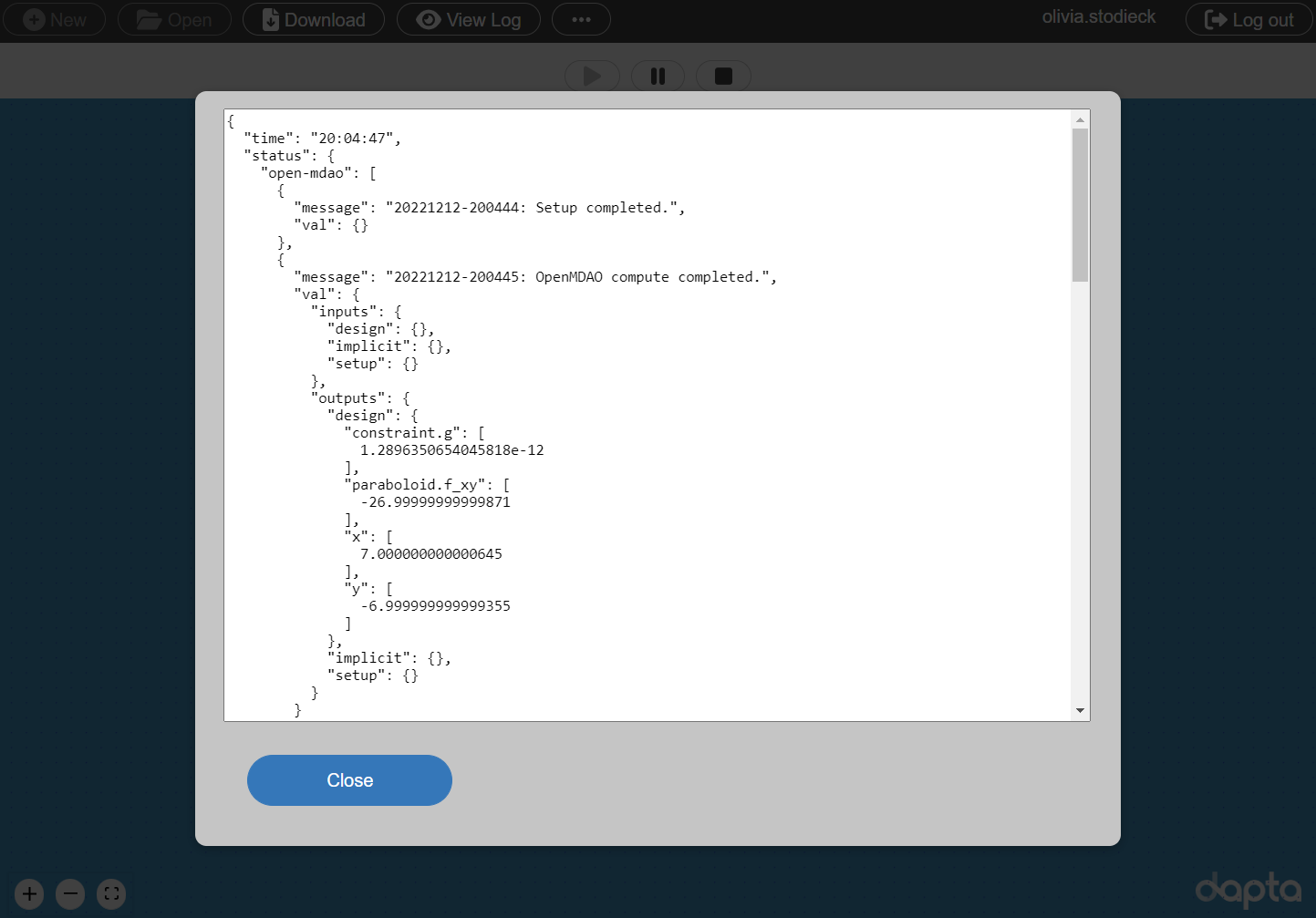Select the open-mdao component in the workspace, open the Log tab and select download files snapshot.

Visually compare the N2 diagram (n2.html) shown below with that from the previous Run.
The new constraint component appears as another Explicit Component under the paraboloid component.

Next, inspect the ‘run_driver.log’ output file. Only 2 iterations, with 2 ‘Function evaluations’ and 2 ‘Gradient evaluations’ were required to reach convergence. The ‘Calling compute_partials’ messages confirm that instead of using finite differencing, we are now requesting the analytical gradient information directly from the paraboloid component. With one paraboloid component evaluation yielding derivatives with respect to all variables, this approach is significantly more efficient than the finite differencing method.

For comparison, a reference pure python/openMDAO implementation of this problem can be found in the Optimization of Paraboloid section of the openMDAO user guide.

Save the session data and Run log by selecting Download from the interface controls.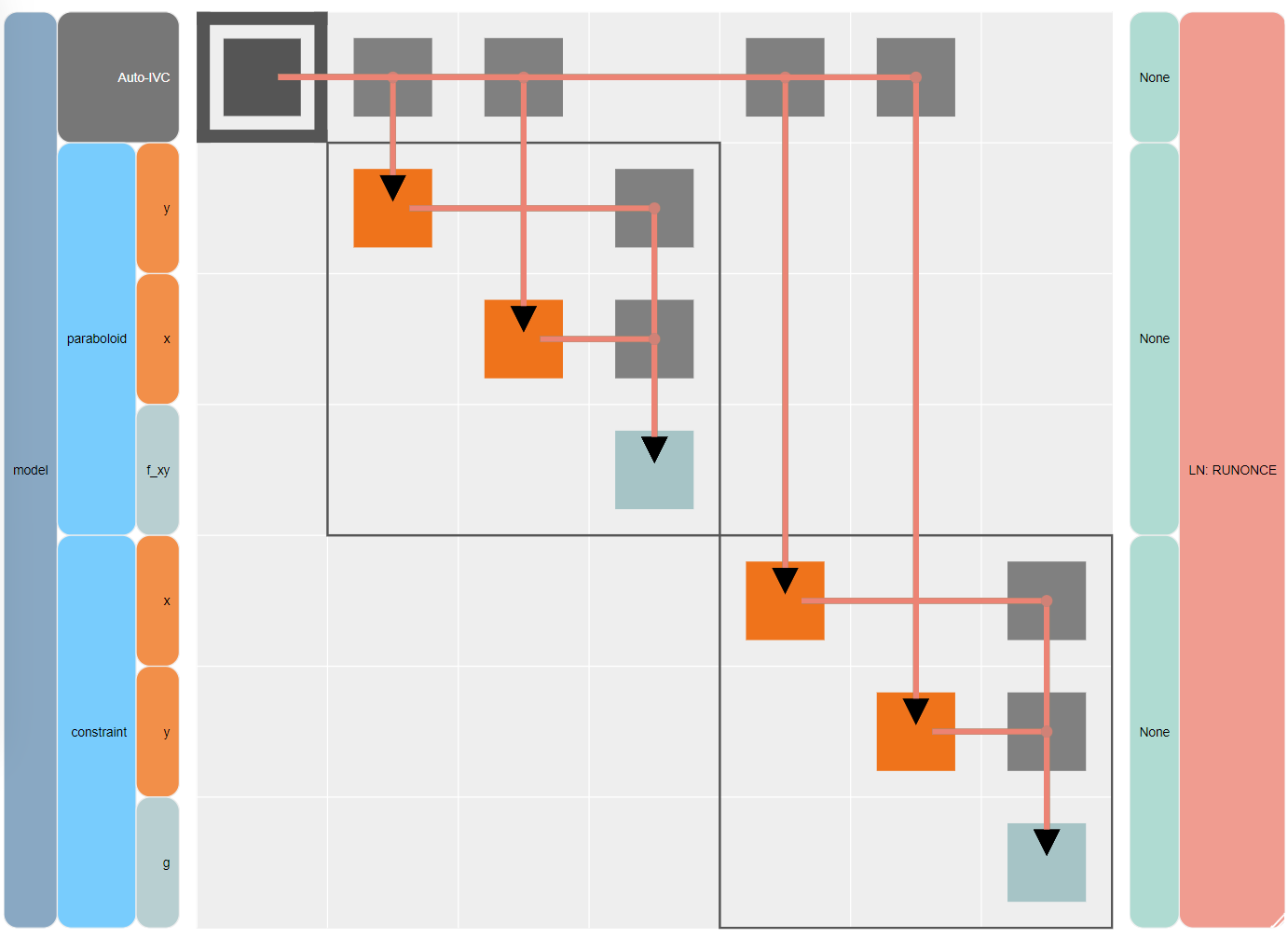## 2.4. Clean-up#

Delete your session by selecting New in the interface. It may take a minute or so for the Cloud session to be reset.

Warning

You should see a warning message whenever you are about to delete a Run. If you select to continue, then all the Run session data (Run log and component logs) will be permanently deleted.